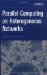# 6.8. A SIMPLE HETEROGENEOUS ALGORITHM SOLVING AN IRREGULAR PROBLEM

## 6.7. SUBNETWORKS

Let us recall once more the fact that a parallel mpC program is a set of parallel processes synchronizing work and interchanging data by means of message passing. The mpC language as it has been introduced allows the programmer to specify the number of processes necessary for parallel solution of the problem, to distribute computations among the processes, and to synchronize their work during the execution of the parallel program. But these means obviously are not sufficient for the specification of data transfer between processes.

So far either all processes of the parallel program or all abstract processors of one or another network took part in the data transfer. The data transfer mainly consisted in broadcasting some value to all participating processes or in gathering values from all participating processes on one of them.

More complicated data transfer, such as that between groups of abstract processors of an mpC network or a parallel exchange between neighboring abstract processors of the network, cannot be specified in the mpC language as it has been presented so far.

The basic mpC device for describing complicated communications is the subnetwork. Any subset of the total set of abstract processors of a network constitutes a subnetwork of this network. For example, any row or column of a network of type Mesh(m,n) is a subnetwork of the network.

Consider the following mpC program:

`#include <mpc.h> #include <string.h> #include <mpc.h> nettype Mesh(int m, int n) {    coord I=m, J=n;    parent [0,0]; }; #define MAXLEN 256 int [*]main() {    net Mesh(2,3) [host]mynet;    [mynet]:    {       char *nodename, me[MAXLEN], neighbor[MAXLEN];       int namelen;       subnet [mynet:I==0]row0, [mynet:I==1]row1;       MPC_Processor_name(&nodename, &namelen);       strcpy(me, nodename);       free(nodename);       [row0]neighbor[] = [row1]me[];       [row1]neighbor[] = [row0]me[];       MPC_Printf("I’m on \"%s\" and have coordinates (%d, %d),\n"           "My neighbor with coordinates"           "(%d, %d) is on \"%s\".\n\n",           me, I coordof mynet, J coordof mynet,           (I coordof mynet + 1)%2,           J coordof mynet, neighbor);    } }`

In this program each abstract processor of network mynet of type Mesh(2,3) outputs to the user’s terminal not only the name of the computer hosting this abstract processor but also the name of the computer that hosts its closest neighbor from other row of abstract processors. In so doing, the program defines two subnetworks row0 and row1 of network mynet. Subnetwork row0 consists of all abstract processors of network mynet whose coordinate I is equal to zero, that is, corresponds to the zeroth processor row of network mynet. This fact is specified with construct [mynet:I==0] before the name of this subnetwork in its definition. Similarly subnetwork row1 corresponds to the first processor row of network mynet.

In general, logical expressions describing abstract processors of subnetworks can be complex leading to the specification of very sophisticated subnetworks. For example, the expression

`I<J && J%2==0`

specifies abstract processors of a network of type Mesh(m,n) that are over the main diagonal of the m n processor arrangement in even columns.

Assignment

`[row0]neighbor[] = [row1]me[]`

is evaluated in parallel by abstract processors of network mynet. During this evaluation each abstract processor of row row1 sends the value of its projection of distributed array me (a vector of MAXLEN characters) to the abstract processor of row row0 with the same coordinate J, where the vector is assigned to the projection of distributed array neighbor (j=0,1,2).

Similarly, during evaluation of assignment

`[row1]neighbor[] = [row0]me[]`

abstract processors of row row0 send in parallel the value of their projection of distributed array me to abstract processors of row row1 with the same coordinate J, where it is assigned to the projection of the distributed array neighbor.

As a result, on the abstract processor with coordinates (0,j), array neighbor will contain the name of the computer, which hosts the abstract processor with coordinates (1,j). On the abstract processor with coordinates (1,j), this array will contain the name of the computer hosting the abstract processor with coordinates (0,j).

The next mpC program demonstrates that the subnetwork can be defined implicitly:

`#include <stdlib.h> #include <string.h> #include <mpc.h> nettype Mesh(int m, int n) {    coord I=m, J=n;    parent [0,0]; }; #define MAXLEN 256 int [*]main() {    net Mesh(2,3) [host]mynet;    [mynet]:    {       char *nodename, me[MAXLEN], neighbor[MAXLEN];       int namelen;       MPC_Processor_name(&nodename, &namelen);       strcpy(me, nodename);       free(nodename);       [mynet:I==0]neighbor[] = [mynet:I==1]me[];       [mynet:I==1]neighbor[] = [mynet:I==0]me[];       MPC_Printf("I’m on \"%s\" and have coordinates (%d, %d),\n"           "My neighbor with coordinates"           "(%d, %d) is on \"%s\".\n\n",           me, I coordof mynet, J coordof mynet,           (I coordof mynet + 1)%2,           J coordof mynet, neighbor);    } } `

The only difference of this program from the previous one is that the row subnetworks are not defined explicitly. In this particular case, usage of implicitly defined subnetworks is justified because it simplifies the program code without a loss of the program efficiency or functionality. But there may be situations where explicit definition of subnetworks cannot be avoided. For example, network functions cannot be called on implicitly defined subnetworks but only on explicitly defined ones.Parallel Computing on Heterogeneous Networks (Wiley Series on Parallel and Distributed Computing)
ISBN: B000W7ZQBO
EAN: N/A
Year: 2005
Pages: 95